### Solution Found!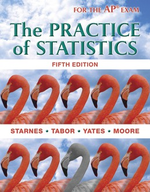# Home computers Refer to Exercise 31. In Jasons SRS, 41 of the students had a computer at

Chapter 9, Problem 35

(choose chapter or problem)

Get Unlimited Answers! Check out our subscriptions
QUESTION:

Refer to Exercise 31. In Jason’s SRS, 41 of the students had a computer at home.

(a) Calculate the test statistic.

(b) Find the P-value using Table A or technology. Show this result as an area under a standard Normal curve.

QUESTION:

Refer to Exercise 31. In Jason’s SRS, 41 of the students had a computer at home.

(a) Calculate the test statistic.

(b) Find the P-value using Table A or technology. Show this result as an area under a standard Normal curve.

Problem 35

Home computers Refer to Exercise 31. In Jason's SRS, 41 of the students had a computer at home.

(a) Calculate the test statistic.

(b) Find the P-value using Table A or technology. Show this result as an area under a standard Normal curve.

Step by Step Solution

Step 1 of 2

(a)

Given,

Sample size, n = 60 (Exercise-31)

The number of success, x = 41

The claimed proportion,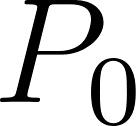= 80% = 0.80.

Then, calculate the sample proportion using the formula, ie;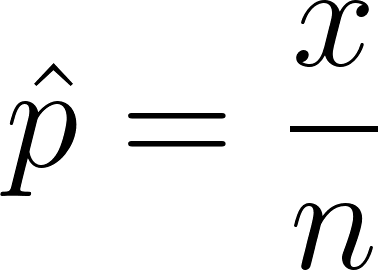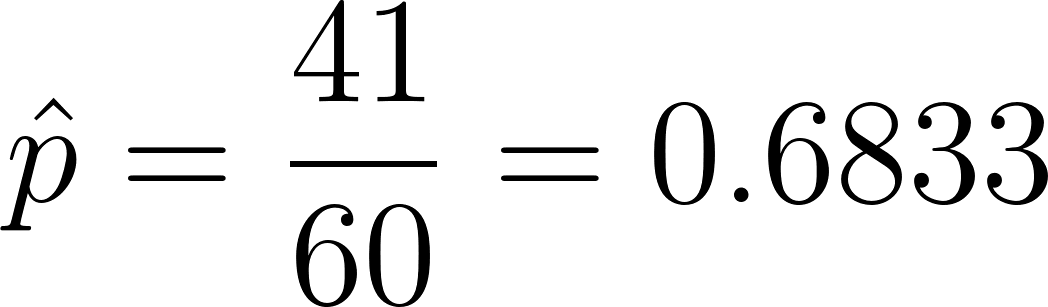Next determine the test statistic,

A test statistic measures how far a sample statistic diverges from what we would expect if the null hypothesiswere true, in standardized units. That is,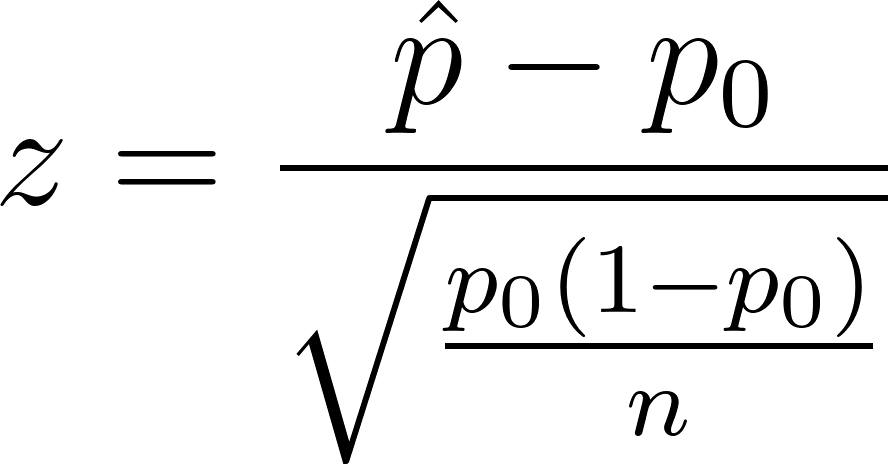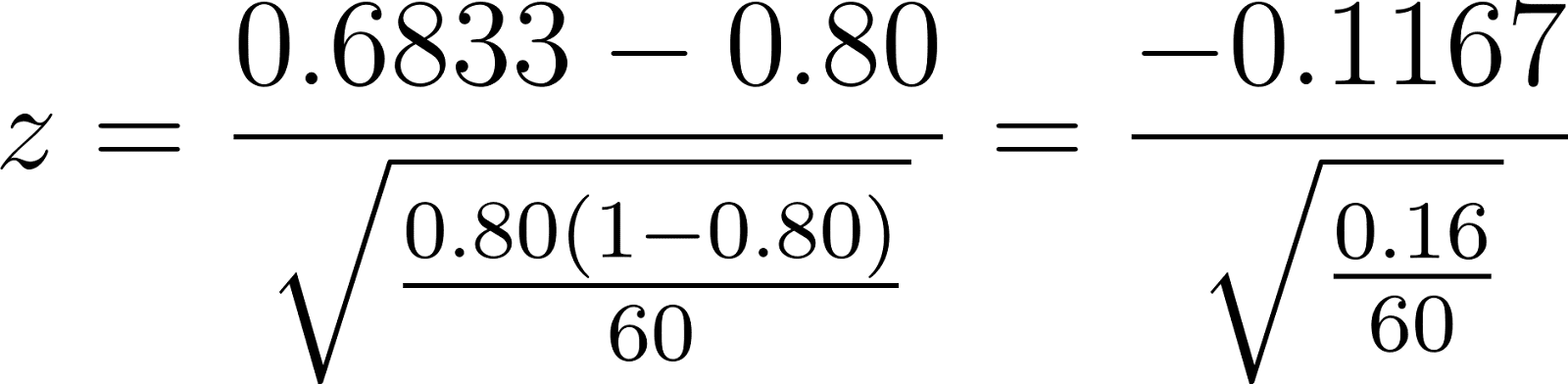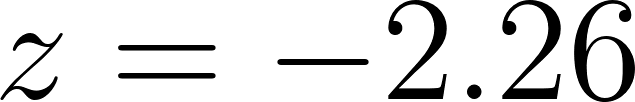The test statistic is -2.26